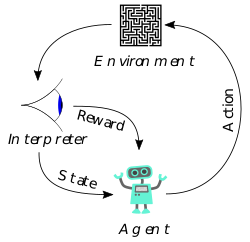# Reinforcement Learning Theory and Examples

Reinforcement learning is a type of machine learning algorithm that allows machines to learn how to achieve the desired outcome by trial and error. The algorithm is based on the principle of operant conditioning, which was first described by psychologist B.F. Skinner in the 1930s.In operant conditioning, an animal is rewarded for completing the desired action (positive reinforcement) or punished for completing an undesired action (negative reinforcement). This process teaches the animal to associate the desired action with a positive or negative outcome, which in turn influences its future behavior.

Reinforcement learning works in a similar way. The algorithm is first given a task, such as steering a car through a maze. It then proceeds to try different actions in order to find the one that leads to the desired outcome (reaching the exit of the maze). The algorithm is “reinforced” each time it completes the task successfully, which encourages it to continue trying new actions.

Reinforcement learning can be used to solve a wide range of problems, from steering a car through a maze to playing a game of chess. It is particularly well-suited to tasks that are too complex for traditional algorithms, such as learning how to walk or speak a new language.### Reinforcement Learning — Theory

Reinforcement learning theory is the study of how agents can learn to maximize rewards through interactions with their environment. The theory is based on the idea of trial and error: agents try different actions and learn which ones lead to the most rewards.

One of the key concepts in reinforcement learning theory is the notion of a reward function. A reward function assigns a value to each action an agent can take, indicating the amount of reward the agent can expect to receive for taking that action. The reward function can be tailored to the specific needs of the agent and can change over time as the agent learns more about the environment.

The most important part of reinforcement learning theory is the learning algorithm, which determines how the agent learns from its experiences. The most common learning algorithm is the so-called Q-learning algorithm, which calculates the value of each activity based on the current state of the environment and the most recent reward the agent received.

One of the advantages of reinforcement learning theory is that agents can learn without any prior knowledge of the environment. This makes reinforcement learning a particularly attractive option for robots and other agents that need to be able to adapt to new environments.

### Some Examples of Reinforcement Learning

One of the most famous examples of reinforcement learning is the game of Go. In Go, the machine must learn how to choose the best move in order to win the game. The game of Go is particularly well-suited for reinforcement learning because it is extremely complex, and there are a huge number of possible moves that the machine could make.A reinforcement learning algorithm can learn how to play Go by gradually increasing its complexity. At first, the machine can be given a set of very simple rules to follow, and then it can be gradually introduced to more complex situations. The machine will learn by trial and error, and it will gradually become better at playing the game.

Reinforcement learning can also be used to learn how to control a robot. In a robot learning scenario, the robot is given a task, such as moving a block from one side of a room to another. The robot will learn how to best complete this task by trial and error.

One of the advantages of reinforcement learning is that it can be used to learn how to solve complex problems that are too difficult for a human to solve. Reinforcement learning algorithms are also able to learn from a large number of examples, which makes them well-suited for problems that are too large or complex for a human to learn from.

### Popular Reinforcement Learning Algorithms

There are a number of different algorithms that can be used for reinforcement learning (RL). We will go over a few of the most popular algorithms below.

First, we have the Q-learning algorithm. This algorithm is a type of model-free RL algorithm, meaning that it does not require a pre-defined model of the environment. The Q-learning algorithm works by learning the optimal action-value function, which is a function that maps each state in the environment to the best possible action to take in that state. The algorithm then uses this function to determine the best action to take in any given state.

Next, we have the SARSA algorithm (State–action–reward–state–action). This algorithm is also a type of model-free RL algorithm. The SARSA algorithm works by learning a policy, which is a function that maps each state in the environment to the best action to take in that state. The algorithm then uses this policy to determine the best action to take in any given state.

Finally, we have the TD learning algorithm (Temporal difference learning). This algorithm is a type of model-based RL algorithm. The TD learning algorithm works by learning a model of the environment. The algorithm then uses this model to determine the best action to take in any given state.

Reinforcement learning is a hot topic in the machine learning community and for good reason. It has shown success in a wide range of domains, from the game playing to robotic control. We’ve seen how reinforcement learning can be used to train agents to play games like Go and poker, as well as navigate complex mazes. In this post, we took a closer look at one particular algorithm used in reinforcement learning called Q-learning. We looked at how the algorithm works and implemented it ourselves using Python. Finally, we applied the algorithm to a simple maze navigation problem.